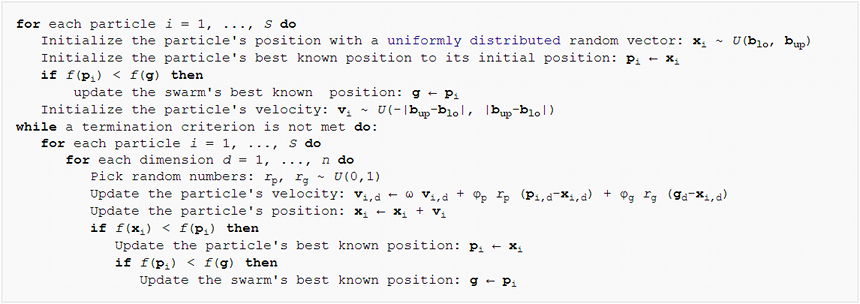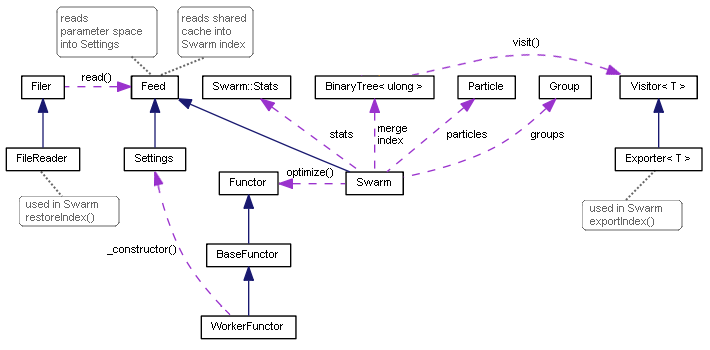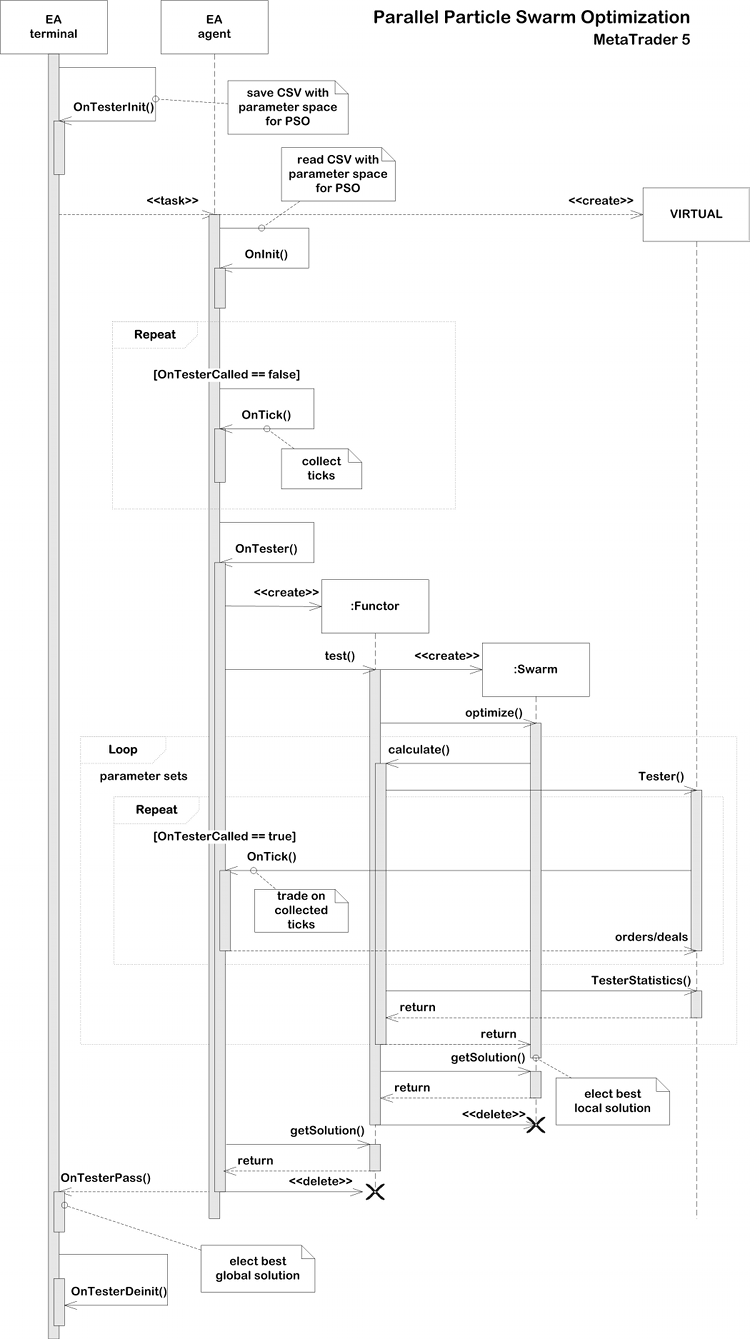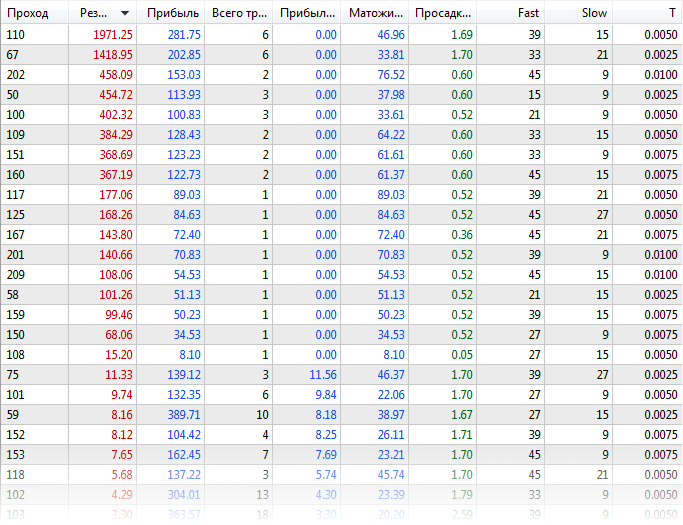# 并行粒子群优化

### 粒子群方法```  class Particle
{
public:
double position[];    // current point
double best[];        // best point known to the particle
double velocity[];    // current speed

double positionValue; // EA performance in current point
double bestValue;     // EA performance in the best point
int    group;

Particle(const int params)
{
ArrayResize(position, params);
ArrayResize(best, params);
ArrayResize(velocity, params);
bestValue = -DBL_MAX;
group = -1;
}
};

```

```  class Group
{
private:
double result;    // best EA performance in the group

public:
double optimum[]; // best known position in the group

Group(const int params)
{
ArrayResize(optimum, params);
ArrayInitialize(optimum, 0);
result = -DBL_MAX;
}

void assign(const double x)
{
result = x;
}

double getResult() const
{
return result;
}

bool isAssigned()
{
return result != -DBL_MAX;
}
};

```

```  class Swarm
{
private:
Particle *particles[];
Group *groups[];
int _size;             // number of particles
int _globals;          // number of groups
int _params;           // number of parameters to optimize

```

```      double highs[];
double lows[];
double steps[];

```

```      double solution[];

```

```    void init(const int size, const int globals, const int params, const double &max[], const double &min[], const double &step[])
{
_size = size;
_globals = globals;
_params = params;

ArrayCopy(highs, max);
ArrayCopy(lows, min);
ArrayCopy(steps, inc);

ArrayResize(solution, _params);

ArrayResize(particles, _size);
for(int i = 0; i < _size; i++)          // loop through particles
{
particles[i] = new Particle(_params);

///do
///{
for(int p = 0; p < _params; p++)    // loop through all dimensions
{
// random placement
particles[i].position[p] = (MathRand() * 1.0 / 32767) * (highs[p] - lows[p]) + lows[p];
// adjust it according to step granularity
if(steps[p] != 0)
{
particles[i].position[p] = ((int)MathRound((particles[i].position[p] - lows[p]) / steps[p])) * steps[p] + lows[p];
}
// the only position is the best so far
particles[i].best[p] = particles[i].position[p];
// random speed
particles[i].velocity[p] = (MathRand() * 1.0 / 32767) * 2 * (highs[p] - lows[p]) - (highs[p] - lows[p]);
}
///}
}

ArrayResize(groups, _globals);
for(int i = 0; i < _globals; i++)
{
groups[i] = new Group(_params);
}

for(int i = 0; i < _size; i++)
{
// random group membership
particles[i].group = (_globals > 1) ?(int)MathMin(MathRand() * 1.0 / 32767 * _globals, _globals - 1) : 0;
}
}

```

```  #define AUTO_SIZE_FACTOR 5

public:
Swarm(const int params, const double &max[], const double &min[], const double &step[])
{
init(params * AUTO_SIZE_FACTOR, (int)MathSqrt(params * AUTO_SIZE_FACTOR), params, max, min, step);
}

Swarm(const int size, const int globals, const int params, const double &max[], const double &min[], const double &step[])
{
init(size, globals, params, max, min, step);
}

```

```    ~Swarm()
{
for(int i = 0; i < _size; i++)
{
delete particles[i];
}
for(int i = 0; i < _globals; i++)
{
delete groups[i];
}
}

```

```    double optimize(Functor &f, const int cycles, const double inertia = 0.8, const double selfBoost = 0.4, const double groupBoost = 0.4)

```

```  class Functor
{
public:
virtual double calculate(const double &vector[]) = 0;
};

```

“optimize”方法的第二个参数是运行算法的最大循环数。以下3个参数设置粒子群优化系数：“inertia”-保持粒子的速度（速度通常随着值小于1而减小），“selfBoost”和“groupBoost”确定粒子在调整其方向到分词/组历史中最已知位置时的响应程度。

```    double optimize(Functor &f, const int cycles, const double inertia = 0.8, const double selfBoost = 0.4, const double groupBoost = 0.4)
{
double result = -DBL_MAX;
ArrayInitialize(solution, 0);

for(int c = 0; c < cycles && !IsStopped(); c++)   // predefined number of cycles
{
for(int i = 0; i < _size && !IsStopped(); i++)  // loop through all particles
{
for(int p = 0; p < _params; p++)              // update particle position and speed
{
double r1 = MathRand() * 1.0 / 32767;
double rg = MathRand() * 1.0 / 32767;
particles[i].velocity[p] = inertia * particles[i].velocity[p] + selfBoost * r1 * (particles[i].best[p] - particles[i].position[p]) + groupBoost * rg * (groups[particles[i].group].optimum[p] - particles[i].position[p]);
particles[i].position[p] = particles[i].position[p] + particles[i].velocity[p];

// make sure to keep the particle inside the boundaries of parameter space
if(particles[i].position[p] < lows[p]) particles[i].position[p] = lows[p];
else if(particles[i].position[p] > highs[p]) particles[i].position[p] = highs[p];

// respect step size
if(steps[p] != 0)
{
particles[i].position[p] = ((int)MathRound((particles[i].position[p] - lows[p]) / steps[p])) * steps[p] + lows[p];
}
}

// get the function value for the particle i
particles[i].positionValue = f.calculate(particles[i].position);

// update the particle's best value and position (if improvement is found)
if(particles[i].positionValue > particles[i].bestValue)
{
particles[i].bestValue = particles[i].positionValue;
ArrayCopy(particles[i].best, particles[i].position);
}

// update the group's best value and position (if improvement is found)
if(particles[i].positionValue > groups[particles[i].group].getResult())
{
groups[particles[i].group].assign(particles[i].positionValue);
ArrayCopy(groups[particles[i].group].optimum, particles[i].position);

// update the global maximum value and solution (if improvement is found)
if(particles[i].positionValue > result)
{
result = particles[i].positionValue;
ArrayCopy(solution, particles[i].position);
}
}
}
}

return result;
}

```

```    bool getSolution(double &result[])
{
ArrayCopy(result, solution);
return !IsStopped();
}

```

### 没有重复的离散世界

Functor 被多次调用以动态地重新计算参数集，但不能保证算法不会多次命中同一点，特别是考虑到沿轴的离散性。为了防止遇到这种巧合情况，有必要以某种方式确定已经计算的点并跳过它们。

```  ulong crc64(ulong crc, const uchar &s[], int l);

```

```  #include <TypeToBytes.mqh>
#include <crc64.mqh>

template<typename T>
ulong crc64(const T &array[])
{
ulong crc = 0;
int len = ArraySize(array);
for(int i = 0; i < len; i++)
{
crc = crc64(crc, _R(array[i]).Bytes, sizeof(T));
}
return crc;
}

```

```  template<typename T>
class TreeNode
{
private:
TreeNode *left;
TreeNode *right;
T value;

public:
TreeNode(T t): value(t) {}
// adds new value into subtrees and returns false or
// returns true if t exists as value of this node or in subtrees
~TreeNode();
TreeNode *getLeft(void) const;
TreeNode *getRight(void) const;
T getValue(void) const;
};

template<typename T>
class BinaryTree
{
private:
TreeNode<T> *root;

public:
~BinaryTree();
};

```

```  class Swarm
{
private:
BinaryTree<ulong> index;

```

“optimize”方法将粒子移动到新位置的部分应该扩展。

```      double optimize(Functor &f, const int cycles, const double inertia = 0.8, const double selfBoost = 0.4, const double groupBoost = 0.4)
{
// ...

double next[];
ArrayResize(next, _params);

for(int c = 0; c < cycles && !IsStopped(); c++)
{
int skipped = 0;
for(int i = 0; i < _size && !IsStopped(); i++)
{
// new placement of particles using temporary array next
for(int p = 0; p < _params; p++)
{
double r1 = MathRand() * 1.0 / 32767;
double rg = MathRand() * 1.0 / 32767;
particles[i].velocity[p] = inertia * particles[i].velocity[p] + selfBoost * r1 * (particles[i].best[p] - particles[i].position[p]) + groupBoost * rg * (groups[particles[i].group].optimum[p] - particles[i].position[p]);
next[p] = particles[i].position[p] + particles[i].velocity[p];
if(next[p] < lows[p]) next[p] = lows[p];
else if(next[p] > highs[p]) next[p] = highs[p];
if(steps[p] != 0)
{
next[p] = ((int)MathRound(next[p] / steps[p])) * steps[p];
}
}

// check if the tree contains this parameter set and add it if not
{
skipped++;
continue;
}

// apply new position to the particle
ArrayCopy(particles[i].position, next);

particles[i].positionValue = f.calculate(particles[i].position);

// ...
}
Print("Cycle ", c, " done, skipped ", skipped, " of ", _size, " / ", result);
if(skipped == _size) break; // full coverage
}

```

### 测试基本功能

```    class BaseFunctor: public Functor
{
protected:
const int params;
double max[], min[], steps[];

public:
BaseFunctor(const int p): params(p) // number of parameters
{
ArrayResize(max, params);
ArrayResize(min, params);
ArrayResize(steps, params);
ArrayInitialize(steps, 0);

PSOTests::register(&this);
}

virtual void test(const int loop)   // worker method
{
Swarm swarm(params, max, min, steps);
swarm.optimize(this, loop);
double result[];
swarm.getSolution(result);
for(int i = 0; i < params; i++)
{
Print(i, " ", result[i]);
}
}
};

```

```  class PSOTests
{
static BaseFunctor *testCases[];

public:
static void register(BaseFunctor *f)
{
int n = ArraySize(testCases);
ArrayResize(testCases, n + 1);
testCases[n] = f;
}

static void run(const int loop = 100)
{
for(int i = 0; i < ArraySize(testCases); i++)
{
testCases[i].test(loop);
}
}
};

```

```      class Sphere: public BaseFunctor
{
public:
Sphere(): BaseFunctor(3) // expected global minimum (0, 0, 0)
{
for(int i = 0; i < params; i++)
{
max[i] = 100;
min[i] = -100;
}
}

virtual void test(const int loop)
{
Print("Optimizing " + typename(this));
BaseFunctor::test(loop);
}

virtual double calculate(const double &vec[])
{
int dim = ArraySize(vec);
double sum = 0;
for(int i = 0; i < dim; i++) sum += pow(vec[i], 2);
return -sum; // negative for maximization
}
};

```

```  void OnStart()
{
PSOTests::Sphere sphere;
PSOTests::Griewank griewank;
PSOTests::Rosenbrock rosenbrock;
PSOTests::run();
}

```

```  Optimizing PSOTests::Sphere
PSO created: 15/3
PSO Processing...
Cycle 0 done, skipped 0 of 15 / -1279.167775306995
Cycle 10 done, skipped 0 of 15 / -231.4807406906516
Cycle 20 done, skipped 0 of 15 / -4.269510657558273
Cycle 30 done, skipped 0 of 15 / -1.931949742316357
Cycle 40 done, skipped 0 of 15 / -0.06018744740061506
Cycle 50 done, skipped 0 of 15 / -0.009498109984732127
Cycle 60 done, skipped 0 of 15 / -0.002058433538555499
Cycle 70 done, skipped 0 of 15 / -0.0001494176502579518
Cycle 80 done, skipped 0 of 15 / -4.141817579039349e-05
Cycle 90 done, skipped 0 of 15 / -1.90930142126799e-05
Cycle 99 done, skipped 0 of 15 / -8.161728746514931e-07
PSO Finished 1500 of 1500 planned calculations: true
0 -0.000594423827318461
1 -0.000484001094843528
2 0.000478096358862763
Optimizing PSOTests::Griewank
PSO created: 10/3
PSO Processing...
Cycle 0 done, skipped 0 of 10 / -26.96927938978973
Cycle 10 done, skipped 0 of 10 / -0.939220906325796
Cycle 20 done, skipped 0 of 10 / -0.3074442362962919
Cycle 30 done, skipped 0 of 10 / -0.121905607345751
Cycle 40 done, skipped 0 of 10 / -0.03294107382891465
Cycle 50 done, skipped 0 of 10 / -0.02138355984774098
Cycle 60 done, skipped 0 of 10 / -0.01060479828529859
Cycle 70 done, skipped 0 of 10 / -0.009728742850384609
Cycle 80 done, skipped 0 of 10 / -0.008640623678293768
Cycle 90 done, skipped 0 of 10 / -0.008578769833161193
Cycle 99 done, skipped 0 of 10 / -0.008578769833161193
PSO Finished 996 of 1000 planned calculations: true
0 3.188612982502877
1 -4.435728146291838
Optimizing PSOTests::Rosenbrock
PSO created: 10/3
PSO Processing...
Cycle 0 done, skipped 0 of 10 / -19.05855349617553
Cycle 10 done, skipped 1 of 10 / -0.4255148824156119
Cycle 20 done, skipped 0 of 10 / -0.1935391314277153
Cycle 30 done, skipped 0 of 10 / -0.006468452482022688
Cycle 40 done, skipped 0 of 10 / -0.001031992354315317
Cycle 50 done, skipped 0 of 10 / -0.00101322411502283
Cycle 60 done, skipped 0 of 10 / -0.0008800704421316765
Cycle 70 done, skipped 0 of 10 / -0.0005593151578155307
Cycle 80 done, skipped 0 of 10 / -0.0005516786893301249
Cycle 90 done, skipped 0 of 10 / -0.0005473814163781119
Cycle 99 done, skipped 0 of 10 / -7.255520122486163e-06
PSO Finished 982 of 1000 planned calculations: true
0 1.001858172119364
1 1.003524791491219

```

### 走向一个并行的世界

```    Swarm(const int size, const int globals, const int params, const double &max[], const double &min[], const double &step[])
{
if(MQLInfoInteger(MQL_OPTIMIZATION))
{
init(size == 0 ? params * AUTO_SIZE_FACTOR : size, 1, params, max, min, step);
}
...

```

```  template<typename T>
class Visitor
{
public:
virtual void visit(TreeNode<T> *node) = 0;
};

```

```  template<typename T>
class Exporter: public Visitor<T>
{
private:
int file;
uint level;

public:
Exporter(const string name): level(0)
{
file = FileOpen(name, FILE_READ | FILE_WRITE | FILE_CSV | FILE_ANSI | FILE_SHARE_READ| FILE_SHARE_WRITE | FILE_COMMON, ',');
}

~Exporter()
{
FileClose(file);
}

virtual void visit(TreeNode<T> *node) override
{
#ifdef PSO_DEBUG_BINTREE
if(node.getLeft()) visit(node.getLeft());
FileWrite(file, node.getValue());
if(node.getRight()) visit(node.getRight());
#else
const T v = node.getValue();
FileWrite(file, v);
level++;
if((level | (uint)v) % 2 == 0)
{
if(node.getLeft()) visit(node.getLeft());
if(node.getRight()) visit(node.getRight());
}
else
{
if(node.getRight()) visit(node.getRight());
if(node.getLeft()) visit(node.getLeft());
}
level--;
#endif
}
};

```

```  template<typename T>
class BinaryTree
{
...
void visit(Visitor<T> *visitor)
{
visitor.visit(root);
}
};

```

Swarm类中的新方法也需要运行该操作。

```    void exportIndex(const int id)
{
const string name = sharedName(id);
Exporter<ulong> exporter(name);
index.visit(&exporter);
}

```

“id”参数表示唯一的过程编号（等于组号）。此参数将用于在测试器中配置优化。exportIndex方法应该在执行两个swarm方法之后立即调用：optimize 和 getSolution。这是由一个调用代码执行的，因为它可能并不总是必需的：我们的第一个“并行”示例（请进一步参阅）并不需要它。如果组的数量等于核心的数量，它们将无法交换任何信息，因为它们将并行启动，并且读取循环中的文件是没有效率的。

exportIndex 中提到的 sharedName 辅助函数允许基于组号、EA名称和终端文件夹创建唯一的名称。

```  #define PPSO_FILE_PREFIX "PPSO-"

string sharedName(const int id, const string prefix = PPSO_FILE_PREFIX, const string ext = ".csv")
{
ushort array[];
StringToShortArray(TerminalInfoString(TERMINAL_PATH), array);
const string program = MQLInfoString(MQL_PROGRAM_NAME) + "-";
if(id != -1)
{
return prefix + program + StringFormat("%08I64X-%04d", crc64(array), id) + ext;
}
return prefix + program + StringFormat("%08I64X-*", crc64(array)) + ext;
}

```

```      bool restoreIndex()
{
string name;
const string filter = sharedName(-1); // use wildcards to merge multiple indices for all cores
long h = FileFindFirst(filter, name, FILE_COMMON);
if(h != INVALID_HANDLE)
{
do
{
}
while(FileFindNext(h, name));
FileFindClose(h);
}
return true;
}

```

```  class Feed
{
public:
virtual bool feed(const int dump) = 0;
};

{
protected:
int dump;

public:
FileReader(const string name, const int flags = 0)
{
dump = FileOpen(name, FILE_READ | FILE_CSV | FILE_SHARE_READ | FILE_SHARE_WRITE | FILE_ANSI | flags, ',');
}

{
return dump != INVALID_HANDLE;
}

{

while(!FileIsEnding(dump))
{
if(!pass.feed(dump))
{
return false;
}
}
return true;
}
};

```

```  class Swarm: public Feed
{
private:
...
int _unique;
int _restored;
BinaryTree<ulong> merge;

public:
...
virtual bool feed(const int dump) override
{
return true;
}
...

```### 并行计算测试

```  enum TEST
{
Sphere,
Griewank,
Rosenbrock
};

sinput int Cycles = 100;
sinput TEST TestCase = Sphere;
sinput int SwarmSize = 0;
input int GroupCount = 0;

```

```  class BaseFunctor: public Functor
{
protected:
const int params;
double max[], min[], steps[];

double optimum;
double result[];

public:
...
virtual void test()
{
Swarm swarm(SwarmSize, GroupCount, params, max, min, steps);
optimum = swarm.optimize(this, Cycles);
swarm.getSolution(result);
}

double getSolution(double &output[]) const
{
ArrayCopy(output, result);
return optimum;
}
};

```

```  double OnTester()
{
MathSrand(GroupCount); // reproducible randomization

BaseFunctor *functor = NULL;

switch(TestCase)
{
case Sphere:
functor = new PSOTests::Sphere();
break;
case Griewank:
functor = new PSOTests::Griewank();
break;
case Rosenbrock:
functor = new PSOTests::Rosenbrock();
break;
}

functor.test();

double output[];
double result = functor.getSolution(output);
if(MQLInfoInteger(MQL_OPTIMIZATION))
{
}
else
{
Print("Solution: ", result);
for(int i = 0; i < ArraySize(output); i++)
{
Print(i, " ", output[i]);
}
}

delete functor;
return result;
}

```

```  int passcount = 0;
double best = -DBL_MAX;
double location[];

void OnTesterPass()
{
ulong pass;
string name;
long id;
double r;
double data[];

while(FrameNext(pass, name, id, r, data))
{
// compare r with all other passes results
if(r > best)
{
best = r;
ArrayCopy(location, data);
}

Print(passcount, " ", id);

const int n = ArraySize(data);
ArrayResize(data, n + 1);
data[n] = r;
ArrayPrint(data, 12);

passcount++;
}
}

void OnTesterDeinit()
{
Print("Solution: ", best);
ArrayPrint(location);
}

```

```  int OnInit()
{
if(!MQLInfoInteger(MQL_TESTER))
{
EventSetTimer(1);
}
return INIT_SUCCEEDED;
}

void OnTimer()
{
EventKillTimer();
OnTester();
}

```

• Cycles — 100;
• TestCase — Griewank;
• SwarmSize — 100;
• GroupCount — 10;

```  Successive PSO of Griewank
PSO created: 100/10
PSO Processing...
Cycle 0 done, skipped 0 of 100 / -1.000317162069485
Cycle 10 done, skipped 0 of 100 / -0.2784790501384311
Cycle 20 done, skipped 0 of 100 / -0.1879188508394087
Cycle 30 done, skipped 0 of 100 / -0.06938172138150922
Cycle 40 done, skipped 0 of 100 / -0.04958694402304631
Cycle 50 done, skipped 0 of 100 / -0.0045818974357138
Cycle 60 done, skipped 0 of 100 / -0.0045818974357138
Cycle 70 done, skipped 0 of 100 / -0.002161613760466419
Cycle 80 done, skipped 0 of 100 / -0.0008991629607246754
Cycle 90 done, skipped 0 of 100 / -1.620636881582982e-05
Cycle 99 done, skipped 0 of 100 / -1.342285474092986e-05
PSO Finished 9948 of 10000 planned calculations: true
Solution: -1.342285474092986e-05
0 0.004966759354110293
1 0.002079707592422949

```

```  Parallel PSO of Griewank
-12.550070232909  -0.002332638407  -0.039510275469
-3.139749741924  4.438437934965 -0.007396077598
3.139620588383  4.438298282495 -0.007396126543
0.000374731767 -0.000072178955 -0.000000071551
Solution: -7.1550806279852e-08 (after 4 passes)
0.00037 -0.00007

```

### EA 交易虚拟化和优化（MetaTrader 5中的 MQL4 API）

```      MqlTick _ticks[];                                     // global array
...                                                   // copy/collect ticks[]
VIRTUAL::Tester(_ticks, OnTick /*, TesterStatistics(STAT_INITIAL_DEPOSIT)*/ );
Print(VIRTUAL::ToString(INT_MAX));                    // output virtual trades in the log
const double result = TesterStatistics(STAT_PROFIT);  // get required performance meter
VIRTUAL::ResetTickets();                              // optional
VIRTUAL::Delete();                                    // optional

```

EA 交易使用解析器引擎根据表达式计算交易信号。其好处将在后面解释。交易策略是相同的：两个移动平均线的交叉，考虑指定的背离阈值。以下是 EA 设置以及信号表达式：

```  input string SignalBuy = "EMA_OPEN_{Fast}(0)/EMA_OPEN_{Slow}(0) > 1 + Threshold";
input string SignalSell = "EMA_OPEN_{Fast}(0)/EMA_OPEN_{Slow}(0) < 1 - Threshold";
input string Variables = "Threshold=0.001";
input int Fast = 10;

input int Slow = 21;

```

• VirtualTester — 启用虚拟测试和/或优化的标志；默认值为false，表示正常操作。
• Estimator — 用于执行优化的变量；默认值为STAT_PROFIT。
• InternalOptimization — 在虚拟模式下启用优化的标志；默认值为false，表示单次虚拟交易。True 就会初始化内部使用 PSO 方法优化。
• PSO_Enable — 启用/禁用 PSO.
• PSO_Cycles — 每个过程中PSO重新计算的周期数；值越大，PSO搜索质量越好，但单个过程在没有反馈（日志记录）的情况下执行的时间更长。
• PSO_SwarmSize — 粒子群大小；默认为0，表示根据参数数量自动进行经验选择。
• PSO_GroupCount — 组数；这是一个用于组织多个过程的增量参数-从0到核心/代理数的值开始，然后增加。
• PSO_RandomSeed — 随机化器；每个组中的组号都被添加到其中，因此它们的初始化方式都不同。

```  class BaseFunctor: public Functor
{
...
public:
virtual bool test(void)
{
Swarm swarm(PSO_SwarmSize, PSO_GroupCount, params, max, min, steps);
if(MQLInfoInteger(MQL_OPTIMIZATION))
{
if(!swarm.restoreIndex()) return false;
}
optimum = swarm.optimize(this, PSO_Cycles);
swarm.getSolution(result);
if(MQLInfoInteger(MQL_OPTIMIZATION))
{
swarm.exportIndex(PSO_GroupCount);
}
return true;
}
};

```

```  class WorkerFunctor: public BaseFunctor
{
string names[];

public:
WorkerFunctor(const Settings &s): BaseFunctor(s.size())
{
s.getNames(names);
for(int i = 0; i < params; i++)
{
max[i] = s.get<double>(i, SET_COLUMN_STOP);
min[i] = s.get<double>(i, SET_COLUMN_START);
steps[i] = s.get<double>(i, SET_COLUMN_STEP);
}
}

virtual double calculate(const double &vec[])
{
VIRTUAL::Tester(_ticks, OnTick, TesterStatistics(STAT_INITIAL_DEPOSIT));
VIRTUAL::ResetTickets();
const double r = TesterStatistics(Estimator);
VIRTUAL::Delete();
return r;
}
};

```

```  #define PPSO_SHARED_SETTINGS __FILE__ + ".csv"
#property tester_file PPSO_SHARED_SETTINGS

```

```  #define MAX_COLUMN 4
#define SET_COLUMN_NAME  0
#define SET_COLUMN_START 1
#define SET_COLUMN_STEP  2
#define SET_COLUMN_STOP  3

```

```  string header[];

void OnTesterInit()
{
int h = FileOpen(PPSO_SHARED_SETTINGS, FILE_ANSI|FILE_WRITE|FILE_CSV, ',');
if(h == INVALID_HANDLE)
{
Print("FileSave error: ", GetLastError());
}

MqlParam parameters[];
string names[];

EXPERT::Parameters(0, parameters, names);
for(int i = 0; i < ArraySize(names); i++)
{
if(ResetOptimizableParam<double>(names[i], h))
{
}
}
FileClose(h); // 5008

bool enabled;
long value, start, step, stop;
if(ParameterGetRange("PSO_GroupCount", enabled, value, start, step, stop))
{
if(!enabled)
{
const int cores = TerminalInfoInteger(TERMINAL_CPU_CORES);
Print("PSO_GroupCount is set to default (number of cores): ", cores);
ParameterSetRange("PSO_GroupCount", true, 0, 1, 1, cores);
}
}

// remove CRC indices from previous optimization runs
Swarm::removeIndex();
}

```

```  template<typename T>
bool ResetOptimizableParam(const string name, const int h)
{
bool enabled;
T value, start, step, stop;
if(ParameterGetRange(name, enabled, value, start, step, stop))
{
// disable all native optimization except for PSO-related params
// preserve original settings in the file h
if((StringFind(name, "PSO_") != 0) && enabled)
{
ParameterSetRange(name, false, value, start, step, stop);
FileWrite(h, name, start, step, stop); // 5007
return true;
}
}
return false;
}

```

```  Settings settings;

int OnInit()
{
...
{
const int n = settings.size();
Print("Got settings: ", n);
}
else
{
if(MQLInfoInteger(MQL_OPTIMIZATION))
{
return INIT_PARAMETERS_INCORRECT;
}
else
{
Print("WARNING!Virtual optimization inside single pass - slowest mode, debugging only");
}
}
...
}

```

```  bool OnTesterCalled = false;

void OnTick()
{
if(VirtualTester && !OnTesterCalled)
{
MqlTick _tick;
SymbolInfoTick(_Symbol, _tick);
const int n = ArraySize(_ticks);
ArrayResize(_ticks, n + 1, n / 2);
_ticks[n] = _tick;

return; // skip all time scope and collect ticks
}
...
}

```

OnTestCalled 变量最初等于false，因此将收集 tick 历史记录。在启动 PSO 之前，在 OnTester 中，晚些时候会把 OnTesterCalled 设为 true。然后 Swarm 对象将开始在循环中计算函子（functor），在循环中调用引用同一个 OnTick 的 VIRTUAL::Tester。这一次，OnTesterCalled 将等于 true，控制权将不转移到 tick 收集模式，而是转移到交易逻辑模式。这将在稍后考虑。将来，随着 PSO 库的进一步发展，通过替换库头文件中的 OnTick 处理程序，可能会出现简化与现有 EA 交易集成的机制。

```  double OnTester()
{
if(VirtualTester)
{
OnTesterCalled = true;

// MQL API implies some limitations for CopyTicksRange function, so ticks are collected in OnTick
const int size = ArraySize(_ticks);
PrintFormat("Ticks size=%d error=%d", size, GetLastError());
if(size <= 0) return 0;

if(settings.isVoid() || !InternalOptimization) // fallback to a single virtual test without PSO
{
VIRTUAL::Tester(_ticks, OnTick, TesterStatistics(STAT_INITIAL_DEPOSIT));
Print(VIRTUAL::ToString(INT_MAX));
return TesterStatistics(Estimator);
}

settings.print();
const int n = settings.size();

if(PSO_Enable)
{
MathSrand(PSO_GroupCount + PSO_RandomSeed); // reproducable randomization
WorkerFunctor worker(settings);
Swarm::Stats stats;
if(worker.test(&stats))
{
double output[];
double result = worker.getSolution(output);
if(MQLInfoInteger(MQL_OPTIMIZATION))
{
FrameAdd(StringFormat("PSO%d/%d", stats.done, stats.planned), PSO_GroupCount, result, output);
}
ArrayResize(output, n + 1);
output[n] = result;
ArrayPrint(output);
return result;
}
}
...
return 0;
}
return TesterStatistics(Estimator);
}

```

OnTesterPass 处理函数与 PPSO test EA 中的处理程序类似，只是帧中接收的数据不是打印到日志中，而是打印到名为 PPSO-EA-name-date_time的 CSV 文件中。```  input string SignalBuy = "EMA_OPEN_{Fast}(0)/EMA_OPEN_{Slow}(0) > 1 + Threshold";
input string SignalSell = "EMA_OPEN_{Fast}(0)/EMA_OPEN_{Slow}(0) < 1 - Threshold";

```

```    const int bar = iBarShift(_Symbol, PERIOD_CURRENT, TimeCurrent());

```

```  input string SignalBuy = "EMA_OPEN_{Fast}(Bar)/EMA_OPEN_{Slow}(Bar) > 1 + Threshold";
input string SignalSell = "EMA_OPEN_{Fast}(Bar)/EMA_OPEN_{Slow}(Bar) < 1 - Threshold";

```

```    const int bar = iBarShift(_Symbol, PERIOD_CURRENT, TimeCurrent()); // NEW
bool sell = p2.with("Bar", bar).resolve();   // WAS: bool sell = p2.resolve();

```

• 完全不要使用指标；不使用任何指标的交易系统在这里有优势；
• 以一种特殊的方式计算指标，独立于EA；许多人使用这种方法，因为这是最快的方法，即使与标准指标相比，也是如此，但它需要很多工作；
• 预先为设置中的所有参数组合创建一组指标；资源密集型；可能需要限制参数范围。

```  input string SignalBuy = "M_EMA_OPEN_9_6_27(Fast,Bar)/M_EMA_OPEN_9_6_27(Slow,Bar) > 1 + T";
input string SignalSell = "M_EMA_OPEN_9_6_27(Fast,Bar)/M_EMA_OPEN_9_6_27(Slow,Bar) < 1 - T";

```

### 实际测试

EURUSD, H1, 范围从2020年起始，使用真实报价点。可以得到以下结果：```  Experts	optimization frame expert ExprBotPSO (EURUSD,H1) processing started
Tester	Experts\ExprBotPSO.ex5 on EURUSD,H1 from 2020.01.01 00:00 to 2020.08.01 00:00
Tester	complete optimization started
...
Core 2	connected
Core 1	connected
Core 2	authorized (agent build 2572)
Core 1	authorized (agent build 2572)
...
Tester	optimization finished, total passes 245
Statistics	optimization done in 20 minutes 55 seconds
Statistics	shortest pass 0:00:05.691, longest pass 0:00:23.114, average pass 0:00:10.206

```

```  Tester	input parameter 'Fast' set to: enable=false, value=9, start=9, step=6, stop=45
Tester	input parameter 'Slow' set to: enable=false, value=21, start=9, step=6, stop=45
Tester	input parameter 'T' set to: enable=false, value=0, start=0, step=0.0025, stop=0.01
Experts	optimization frame expert ExprBotPSO (EURUSD,H1) processing started
Tester	Experts\ExprBotPSO.ex5 on EURUSD,H1 from 2020.01.01 00:00 to 2020.08.01 00:00
Tester	complete optimization started
...
Core 1	connected
Core 2	connected
Core 2	authorized (agent build 2572)
Core 1	authorized (agent build 2572)
...
Tester	optimization finished, total passes 4
Statistics	optimization done in 4 minutes 00 seconds
Statistics	shortest pass 0:01:27.723, longest pass 0:02:24.841, average pass 0:01:56.597
Statistics	4 frames (784 bytes total, 196 bytes per frame) received

```

```  22:22:52.261	ExprBotPSO (EURUSD,H1)	2 tmp-files deleted
22:25:07.981	ExprBotPSO (EURUSD,H1)	0 PSO75/1500 0 1974.400000000025
22:25:23.348	ExprBotPSO (EURUSD,H1)	2 PSO84/1500 2 402.6000000000062
22:26:51.165	ExprBotPSO (EURUSD,H1)	3 PSO70/1500 3 455.000000000003
22:26:52.451	ExprBotPSO (EURUSD,H1)	1 PSO79/1500 1 458.3000000000047
22:26:52.466	ExprBotPSO (EURUSD,H1)	Solution: 1974.400000000025
22:26:52.466	ExprBotPSO (EURUSD,H1)	39.00000 15.00000  0.00500

```

• 接近市场价格的限价订单可能通过不同的方式触发
• 保证金未计算或计算不太准确
• 佣金不会自动计算（MQL API限制），但可以通过其他输入参数进行编程
• 在净额结算模式下，订单和交易的账户处理可能有所不同
• 只支持当前交易品种

```  Ticks size=15060113 error=0
[,0]     [,1]     [,2]     [,3]
[0,] "Fast"   "9"      "6"      "45"
[1,] "Slow"   "9"      "6"      "45"
[2,] "T"      "0"      "0.0025" "0.01"
PSO created: 15/3
PSO Processing...
Fast:9.0, Slow:33.0, T:0.0025, 1.31285
Fast:21.0, Slow:21.0, T:0.0025, -1.0
Fast:15.0, Slow:33.0, T:0.0075, -1.0
Fast:27.0, Slow:39.0, T:0.0025, 0.07673
Fast:9.0, Slow:9.0, T:0.005, -1.0
Fast:33.0, Slow:21.0, T:0.01, -1.0
Fast:39.0, Slow:45.0, T:0.0025, -1.0
Fast:15.0, Slow:15.0, T:0.0025, -1.0
Fast:33.0, Slow:21.0, T:0.0, 0.32895
Fast:33.0, Slow:39.0, T:0.0075, -1.0
Fast:33.0, Slow:15.0, T:0.005, 384.5
Fast:15.0, Slow:27.0, T:0.0, 2.44486
Fast:39.0, Slow:27.0, T:0.0025, 11.41199
Fast:9.0, Slow:15.0, T:0.0, 1.08838
Fast:33.0, Slow:27.0, T:0.0075, -1.0
Cycle 0 done, skipped 0 of 15 / 384.5000000000009
...
Fast:45.0, Slow:9.0, T:0.0025, 0.86209
Fast:21.0, Slow:15.0, T:0.005, -1.0
Cycle 15 done, skipped 13 of 15 / 402.6000000000062
Fast:21.0, Slow:15.0, T:0.0025, 101.4
Cycle 16 done, skipped 14 of 15 / 402.6000000000062
Fast:27.0, Slow:15.0, T:0.0025, 8.18754
Fast:39.0, Slow:15.0, T:0.005, 1974.40002
Cycle 17 done, skipped 13 of 15 / 1974.400000000025
Fast:45.0, Slow:9.0, T:0.005, 1.00344
Cycle 18 done, skipped 14 of 15 / 1974.400000000025
Cycle 19 done, skipped 15 of 15 / 1974.400000000025
PSO Finished 89 of 1500 planned calculations: true
39.00000   15.00000    0.00500 1974.40000
final balance 10000.00 USD
OnTester result 1974.400000000025

```

### MQL5 API EA 交易的虚拟化

```  #include <fxsaber/Virtual/Virtual.mqh>
#include <MT5Bridge.mqh>

#include <Expert\Expert.mqh>
#include <Expert\Signal\SignalMA.mqh>
#include <Expert\Trailing\TrailingParabolicSAR.mqh>
#include <Expert\Money\MoneySizeOptimized.mqh>
...

```

MT5Bridge.mqh 因为它的功能没有经过广泛的测试，所以处于实验状态。这是一个概念研究的证明。您可以使用源代码进行调试和 bug 修复。

## 量化课程

移动端课程